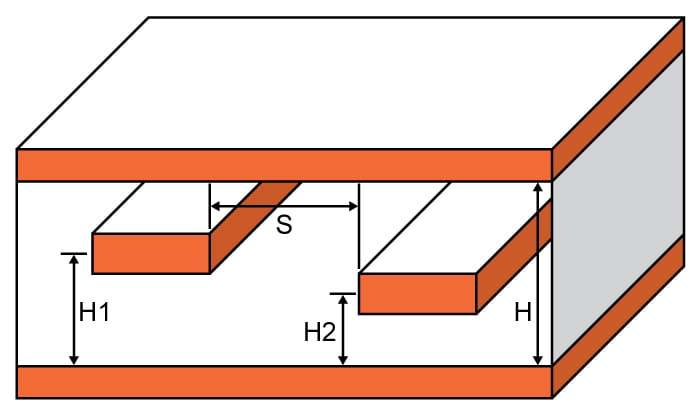# Stripline Crosstalk Calculator

## This tool is designed to calculate cross-talk coefficient and coupled voltage for a stripline

ns
Volts

dB
Volts

### Overview

This calculator is designed to determine the magnitude of the crosstalk on a stripline if its dimensions and source voltage is provided. The magnitude of the coupled voltage between adjacent traces is also provided.

In radio technology, crosstalk is a phenomenon wherein a signal in a conductor jumps to an adjacent conductor. Crosstalk is unwanted and there are measures to prevent it.### Equations

$$T_{RT} = 1.017\sqrt{\varepsilon _{r\cdot 0.475+0.67}}\cdot L\cdot 2$$

$$S_{eff}=\sqrt{S^{2}+\left (h_{2}-h_{1} \right )^{2}}$$

$$h_{1eff}=\frac{h_{1}\cdot \left ( H-h_{1} \right )}{h_{1}+ \left ( H- h_{1} \right )}$$

$$h_{2eff}=\frac{h_{2}\cdot \left ( H-h_{2} \right )}{h_{2}+ \left ( H- h_{2} \right )}$$

if,

$$\frac{T_{RT}}{T_{R}}\leq 1$$

then,

$$CT_{dB} = 20\log \left ( \frac{1}{1+\left ( \frac{ S_{eff}^{2}}{h_{1eff}\cdot h_{2eff} } \right )}\cdot \frac{T_{RT}}{T_{R}} \right )$$
$$V_{crosstalk} = V\cdot \frac{1}{1+\left ( \frac{S_{eff}^{2}}{h_{1eff}\cdot h_{2eff} } \right )}\cdot \frac{T_{RT}}{T_{R}}$$

else,

$$CT_{dB} = 20\log \left ( \frac{1}{1+\left ( \frac{ S_{eff}^{2}}{ h_{1eff}\cdot h_{2eff}} \right )^{2}}\right )$$
$$V_{crosstalk} = V\cdot \frac{1}{1+\left ( \frac{ S_{eff}^{2}}{ h_{1eff}\cdot h_{2eff}} \right )^{2}}$$